» Code examples / Generative Deep Learning / Vector-Quantized Variational Autoencoders

# Vector-Quantized Variational Autoencoders

Author: Sayak Paul
Date created: 2021/07/21View in ColabGitHub source

Description: Training a VQ-VAE for image reconstruction and codebook sampling for generation.

In this example, we develop a Vector Quantized Variational Autoencoder (VQ-VAE). VQ-VAE was proposed in Neural Discrete Representation Learning by van der Oord et al. In standard VAEs, the latent space is continuous and is sampled from a Gaussian distribution. It is generally harder to learn such a continuous distribution via gradient descent. VQ-VAEs, on the other hand, operate on a discrete latent space, making the optimization problem simpler. It does so by maintaining a discrete codebook. The codebook is developed by discretizing the distance between continuous embeddings and the encoded outputs. These discrete code words are then fed to the decoder, which is trained to generate reconstructed samples.

For an overview of VQ-VAEs, please refer to the original paper and this video explanation. If you need a refresher on VAEs, you can refer to this book chapter. VQ-VAEs are one of the main recipes behind DALL-E and the idea of a codebook is used in VQ-GANs.

This example uses implementation details from the official VQ-VAE tutorial from DeepMind.

## Requirements

To run this example, you will need TensorFlow 2.5 or higher, as well as TensorFlow Probability, which can be installed using the command below.

``````!pip install -q tensorflow-probability
``````

## Imports

``````import numpy as np
import matplotlib.pyplot as plt

from tensorflow import keras
from tensorflow.keras import layers
import tensorflow_probability as tfp
import tensorflow as tf
``````

## `VectorQuantizer` layer

First, we implement a custom layer for the vector quantizer, which is the layer in between the encoder and decoder. Consider an output from the encoder, with shape ```(batch_size, height, width, num_filters)```. The vector quantizer will first flatten this output, only keeping the `num_filters` dimension intact. So, the shape would become ```(batch_size * height * width, num_filters)```. The rationale behind this is to treat the total number of filters as the size for the latent embeddings.

An embedding table is then initialized to learn a codebook. We measure the L2-normalized distance between the flattened encoder outputs and code words of this codebook. We take the code that yields the minimum distance, and we apply one-hot encoding to achieve quantization. This way, the code yielding the minimum distance to the corresponding encoder output is mapped as one and the remaining codes are mapped as zeros.

Since the quantization process is not differentiable, we apply a straight-through estimator in between the decoder and the encoder, so that the decoder gradients are directly propagated to the encoder. As the encoder and decoder share the same channel space, the decoder gradients are still meaningful to the encoder.

``````class VectorQuantizer(layers.Layer):
def __init__(self, num_embeddings, embedding_dim, beta=0.25, **kwargs):
super().__init__(**kwargs)
self.embedding_dim = embedding_dim
self.num_embeddings = num_embeddings

# The `beta` parameter is best kept between [0.25, 2] as per the paper.
self.beta = beta

# Initialize the embeddings which we will quantize.
w_init = tf.random_uniform_initializer()
self.embeddings = tf.Variable(
initial_value=w_init(
shape=(self.embedding_dim, self.num_embeddings), dtype="float32"
),
trainable=True,
name="embeddings_vqvae",
)

def call(self, x):
# Calculate the input shape of the inputs and
# then flatten the inputs keeping `embedding_dim` intact.
input_shape = tf.shape(x)
flattened = tf.reshape(x, [-1, self.embedding_dim])

# Quantization.
encoding_indices = self.get_code_indices(flattened)
encodings = tf.one_hot(encoding_indices, self.num_embeddings)
quantized = tf.matmul(encodings, self.embeddings, transpose_b=True)

# Reshape the quantized values back to the original input shape
quantized = tf.reshape(quantized, input_shape)

# https://keras.io/guides/making_new_layers_and_models_via_subclassing/. Check
# the original paper to get a handle on the formulation of the loss function.
commitment_loss = tf.reduce_mean((tf.stop_gradient(quantized) - x) ** 2)
codebook_loss = tf.reduce_mean((quantized - tf.stop_gradient(x)) ** 2)

# Straight-through estimator.
quantized = x + tf.stop_gradient(quantized - x)
return quantized

def get_code_indices(self, flattened_inputs):
# Calculate L2-normalized distance between the inputs and the codes.
similarity = tf.matmul(flattened_inputs, self.embeddings)
distances = (
tf.reduce_sum(flattened_inputs ** 2, axis=1, keepdims=True)
+ tf.reduce_sum(self.embeddings ** 2, axis=0)
- 2 * similarity
)

# Derive the indices for minimum distances.
encoding_indices = tf.argmin(distances, axis=1)
return encoding_indices
``````

A note on straight-through estimation:

This line of code does the straight-through estimation part: ```quantized = x + tf.stop_gradient(quantized - x)```. During backpropagation, `(quantized - x)` won't be included in the computation graph and the gradients obtained for `quantized` will be copied for `inputs`. Thanks to this video for helping me understand this technique.

## Encoder and decoder

Now for the encoder and the decoder for the VQ-VAE. We will keep them small so that their capacity is a good fit for the MNIST dataset. The implementation of the encoder and decoder come from this example.

Note that activations other than ReLU may not work for the encoder and decoder layers in the quantization architecture: Leaky ReLU activated layers, for example, have proven difficult to train, resulting in intermittent loss spikes that the model has trouble recovering from.

``````def get_encoder(latent_dim=16):
encoder_inputs = keras.Input(shape=(28, 28, 1))
x = layers.Conv2D(32, 3, activation="relu", strides=2, padding="same")(
encoder_inputs
)
x = layers.Conv2D(64, 3, activation="relu", strides=2, padding="same")(x)
return keras.Model(encoder_inputs, encoder_outputs, name="encoder")

def get_decoder(latent_dim=16):
latent_inputs = keras.Input(shape=get_encoder(latent_dim).output.shape[1:])
x = layers.Conv2DTranspose(64, 3, activation="relu", strides=2, padding="same")(
latent_inputs
)
x = layers.Conv2DTranspose(32, 3, activation="relu", strides=2, padding="same")(x)
return keras.Model(latent_inputs, decoder_outputs, name="decoder")
``````

## Standalone VQ-VAE model

``````def get_vqvae(latent_dim=16, num_embeddings=64):
vq_layer = VectorQuantizer(num_embeddings, latent_dim, name="vector_quantizer")
encoder = get_encoder(latent_dim)
decoder = get_decoder(latent_dim)
inputs = keras.Input(shape=(28, 28, 1))
encoder_outputs = encoder(inputs)
quantized_latents = vq_layer(encoder_outputs)
reconstructions = decoder(quantized_latents)
return keras.Model(inputs, reconstructions, name="vq_vae")

get_vqvae().summary()
``````
``````Model: "vq_vae"
_________________________________________________________________
Layer (type)                 Output Shape              Param #
=================================================================
input_4 (InputLayer)         [(None, 28, 28, 1)]       0
_________________________________________________________________
encoder (Functional)         (None, 7, 7, 16)          19856
_________________________________________________________________
vector_quantizer (VectorQuan (None, 7, 7, 16)          1024
_________________________________________________________________
decoder (Functional)         (None, 28, 28, 1)         28033
=================================================================
Total params: 48,913
Trainable params: 48,913
Non-trainable params: 0
_________________________________________________________________
``````

Note that the output channels of the encoder should match the `latent_dim` for the vector quantizer.

## Wrapping up the training loop inside `VQVAETrainer`

``````class VQVAETrainer(keras.models.Model):
def __init__(self, train_variance, latent_dim=32, num_embeddings=128, **kwargs):
super().__init__(**kwargs)
self.train_variance = train_variance
self.latent_dim = latent_dim
self.num_embeddings = num_embeddings

self.vqvae = get_vqvae(self.latent_dim, self.num_embeddings)

self.total_loss_tracker = keras.metrics.Mean(name="total_loss")
self.reconstruction_loss_tracker = keras.metrics.Mean(
name="reconstruction_loss"
)
self.vq_loss_tracker = keras.metrics.Mean(name="vq_loss")

@property
def metrics(self):
return [
self.total_loss_tracker,
self.reconstruction_loss_tracker,
self.vq_loss_tracker,
]

def train_step(self, x):
# Outputs from the VQ-VAE.
reconstructions = self.vqvae(x)

# Calculate the losses.
reconstruction_loss = (
tf.reduce_mean((x - reconstructions) ** 2) / self.train_variance
)
total_loss = reconstruction_loss + sum(self.vqvae.losses)

# Backpropagation.

# Loss tracking.
self.total_loss_tracker.update_state(total_loss)
self.reconstruction_loss_tracker.update_state(reconstruction_loss)
self.vq_loss_tracker.update_state(sum(self.vqvae.losses))

# Log results.
return {
"loss": self.total_loss_tracker.result(),
"reconstruction_loss": self.reconstruction_loss_tracker.result(),
"vqvae_loss": self.vq_loss_tracker.result(),
}
``````

## Load and preprocess the MNIST dataset

``````(x_train, _), (x_test, _) = keras.datasets.mnist.load_data()

x_train = np.expand_dims(x_train, -1)
x_test = np.expand_dims(x_test, -1)
x_train_scaled = (x_train / 255.0) - 0.5
x_test_scaled = (x_test / 255.0) - 0.5

data_variance = np.var(x_train / 255.0)
``````
``````Downloading data from https://storage.googleapis.com/tensorflow/tf-keras-datasets/mnist.npz
11493376/11490434 [==============================] - 0s 0us/step
``````

## Train the VQ-VAE model

``````vqvae_trainer = VQVAETrainer(data_variance, latent_dim=16, num_embeddings=128)
vqvae_trainer.fit(x_train_scaled, epochs=30, batch_size=128)
``````
``````Epoch 1/30
469/469 [==============================] - 18s 6ms/step - loss: 2.2962 - reconstruction_loss: 0.3869 - vqvae_loss: 1.5950
Epoch 2/30
469/469 [==============================] - 3s 6ms/step - loss: 2.2980 - reconstruction_loss: 0.1692 - vqvae_loss: 2.1108
Epoch 3/30
469/469 [==============================] - 3s 6ms/step - loss: 1.1356 - reconstruction_loss: 0.1281 - vqvae_loss: 0.9997
Epoch 4/30
469/469 [==============================] - 3s 6ms/step - loss: 0.6112 - reconstruction_loss: 0.1030 - vqvae_loss: 0.5031
Epoch 5/30
469/469 [==============================] - 3s 6ms/step - loss: 0.4375 - reconstruction_loss: 0.0883 - vqvae_loss: 0.3464
Epoch 6/30
469/469 [==============================] - 3s 6ms/step - loss: 0.3579 - reconstruction_loss: 0.0788 - vqvae_loss: 0.2775
Epoch 7/30
469/469 [==============================] - 3s 5ms/step - loss: 0.3197 - reconstruction_loss: 0.0725 - vqvae_loss: 0.2457
Epoch 8/30
469/469 [==============================] - 3s 5ms/step - loss: 0.2960 - reconstruction_loss: 0.0673 - vqvae_loss: 0.2277
Epoch 9/30
469/469 [==============================] - 3s 5ms/step - loss: 0.2798 - reconstruction_loss: 0.0640 - vqvae_loss: 0.2152
Epoch 10/30
469/469 [==============================] - 3s 5ms/step - loss: 0.2681 - reconstruction_loss: 0.0612 - vqvae_loss: 0.2061
Epoch 11/30
469/469 [==============================] - 3s 6ms/step - loss: 0.2578 - reconstruction_loss: 0.0590 - vqvae_loss: 0.1986
Epoch 12/30
469/469 [==============================] - 3s 6ms/step - loss: 0.2551 - reconstruction_loss: 0.0574 - vqvae_loss: 0.1974
Epoch 13/30
469/469 [==============================] - 3s 6ms/step - loss: 0.2526 - reconstruction_loss: 0.0560 - vqvae_loss: 0.1961
Epoch 14/30
469/469 [==============================] - 3s 6ms/step - loss: 0.2485 - reconstruction_loss: 0.0546 - vqvae_loss: 0.1936
Epoch 15/30
469/469 [==============================] - 3s 6ms/step - loss: 0.2462 - reconstruction_loss: 0.0533 - vqvae_loss: 0.1926
Epoch 16/30
469/469 [==============================] - 3s 6ms/step - loss: 0.2445 - reconstruction_loss: 0.0523 - vqvae_loss: 0.1920
Epoch 17/30
469/469 [==============================] - 3s 6ms/step - loss: 0.2427 - reconstruction_loss: 0.0515 - vqvae_loss: 0.1911
Epoch 18/30
469/469 [==============================] - 3s 6ms/step - loss: 0.2405 - reconstruction_loss: 0.0505 - vqvae_loss: 0.1898
Epoch 19/30
469/469 [==============================] - 3s 6ms/step - loss: 0.2368 - reconstruction_loss: 0.0495 - vqvae_loss: 0.1871
Epoch 20/30
469/469 [==============================] - 3s 5ms/step - loss: 0.2310 - reconstruction_loss: 0.0486 - vqvae_loss: 0.1822
Epoch 21/30
469/469 [==============================] - 3s 5ms/step - loss: 0.2245 - reconstruction_loss: 0.0475 - vqvae_loss: 0.1769
Epoch 22/30
469/469 [==============================] - 3s 5ms/step - loss: 0.2205 - reconstruction_loss: 0.0469 - vqvae_loss: 0.1736
Epoch 23/30
469/469 [==============================] - 3s 5ms/step - loss: 0.2195 - reconstruction_loss: 0.0465 - vqvae_loss: 0.1730
Epoch 24/30
469/469 [==============================] - 3s 5ms/step - loss: 0.2187 - reconstruction_loss: 0.0461 - vqvae_loss: 0.1726
Epoch 25/30
469/469 [==============================] - 3s 5ms/step - loss: 0.2180 - reconstruction_loss: 0.0458 - vqvae_loss: 0.1721
Epoch 26/30
469/469 [==============================] - 3s 5ms/step - loss: 0.2163 - reconstruction_loss: 0.0454 - vqvae_loss: 0.1709
Epoch 27/30
469/469 [==============================] - 3s 5ms/step - loss: 0.2156 - reconstruction_loss: 0.0452 - vqvae_loss: 0.1704
Epoch 28/30
469/469 [==============================] - 3s 5ms/step - loss: 0.2146 - reconstruction_loss: 0.0449 - vqvae_loss: 0.1696
Epoch 29/30
469/469 [==============================] - 3s 5ms/step - loss: 0.2139 - reconstruction_loss: 0.0447 - vqvae_loss: 0.1692
Epoch 30/30
469/469 [==============================] - 3s 5ms/step - loss: 0.2127 - reconstruction_loss: 0.0444 - vqvae_loss: 0.1682

<tensorflow.python.keras.callbacks.History at 0x7f96402f4e50>
``````

## Reconstruction results on the test set

``````def show_subplot(original, reconstructed):
plt.subplot(1, 2, 1)
plt.imshow(original.squeeze() + 0.5)
plt.title("Original")
plt.axis("off")

plt.subplot(1, 2, 2)
plt.imshow(reconstructed.squeeze() + 0.5)
plt.title("Reconstructed")
plt.axis("off")

plt.show()

trained_vqvae_model = vqvae_trainer.vqvae
idx = np.random.choice(len(x_test_scaled), 10)
test_images = x_test_scaled[idx]
reconstructions_test = trained_vqvae_model.predict(test_images)

for test_image, reconstructed_image in zip(test_images, reconstructions_test):
show_subplot(test_image, reconstructed_image)
``````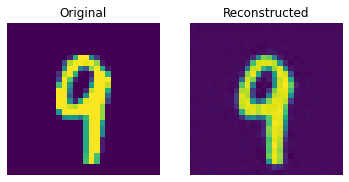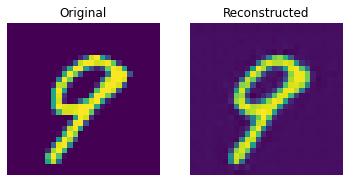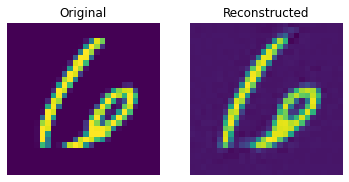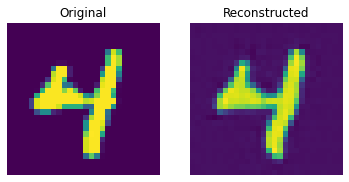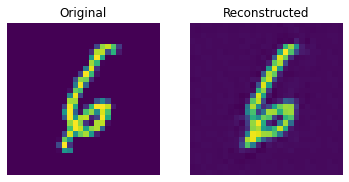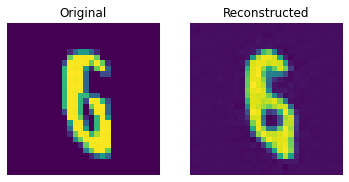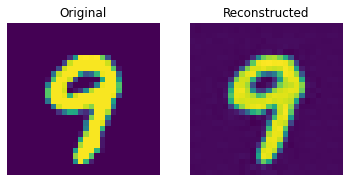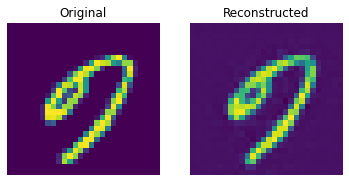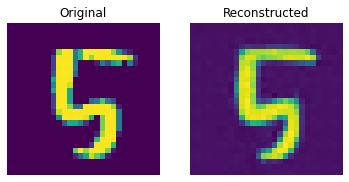These results look decent. You are encouraged to play with different hyperparameters (especially the number of embeddings and the dimensions of the embeddings) and observe how they affect the results.

## Visualizing the discrete codes

``````encoder = vqvae_trainer.vqvae.get_layer("encoder")
quantizer = vqvae_trainer.vqvae.get_layer("vector_quantizer")

encoded_outputs = encoder.predict(test_images)
flat_enc_outputs = encoded_outputs.reshape(-1, encoded_outputs.shape[-1])
codebook_indices = quantizer.get_code_indices(flat_enc_outputs)
codebook_indices = codebook_indices.numpy().reshape(encoded_outputs.shape[:-1])

for i in range(len(test_images)):
plt.subplot(1, 2, 1)
plt.imshow(test_images[i].squeeze() + 0.5)
plt.title("Original")
plt.axis("off")

plt.subplot(1, 2, 2)
plt.imshow(codebook_indices[i])
plt.title("Code")
plt.axis("off")
plt.show()
``````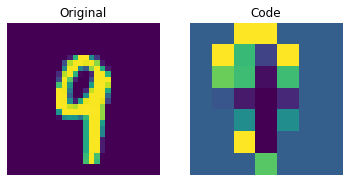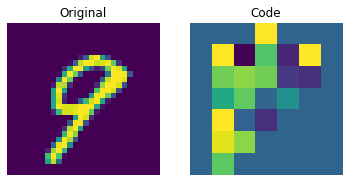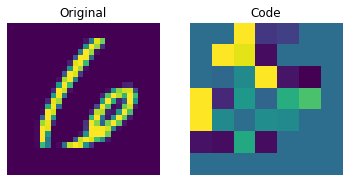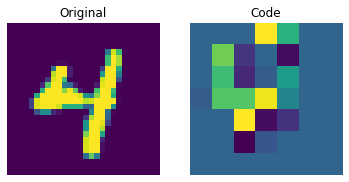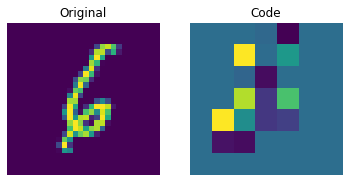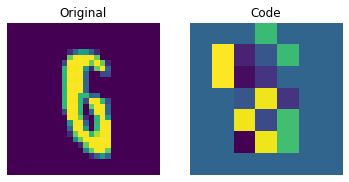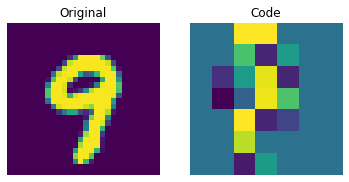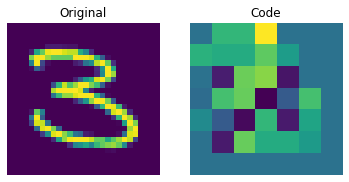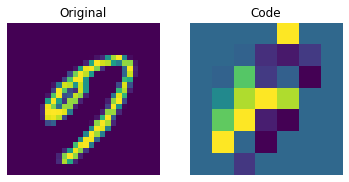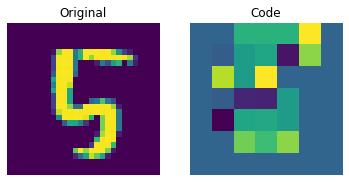The figure above shows that the discrete codes have been able to capture some regularities from the dataset. Now, how do we sample from this codebook to create novel images? Since these codes are discrete and we imposed a categorical distribution on them, we cannot use them yet to generate anything meaningful until we can generate likely sequences of codes that we can give to the decoder. The authors use a PixelCNN to train these codes so that they can be used as powerful priors to generate novel examples. PixelCNN was proposed in Conditional Image Generation with PixelCNN Decoders by van der Oord et al. We will borrow code from this example by van der Oord et al. We borrow the implementation from this PixelCNN example. It's an autoregressive generative model where the outputs are conditional on the prior ones. In other words, a PixelCNN generates an image on a pixel-by-pixel basis. For the purpose in this example, however, its task is to generate code book indices instead of pixels directly. The trained VQ-VAE decoder is used to map the indices generated by the PixelCNN back into the pixel space.

## PixelCNN hyperparameters

``````num_residual_blocks = 2
num_pixelcnn_layers = 2
pixelcnn_input_shape = encoded_outputs.shape[1:-1]
print(f"Input shape of the PixelCNN: {pixelcnn_input_shape}")
``````
``````Input shape of the PixelCNN: (7, 7)
``````

This input shape represents the reduction in the resolution performed by the encoder. With "same" padding, this exactly halves the "resolution" of the output shape for each stride-2 convolution layer. So, with these two layers, we end up with an encoder output tensor of 7x7 on axes 2 and 3, with the first axis as the batch size and the last axis being the code book embedding size. Since the quantization layer in the autoencoder maps these 7x7 tensors to indices of the code book, these output layer axis sizes must be matched by the PixelCNN as the input shape. The task of the PixelCNN for this architecture is to generate likely 7x7 arrangements of codebook indices.

Note that this shape is something to optimize for in larger-sized image domains, along with the code book sizes. Since the PixelCNN is autoregressive, it needs to pass over each codebook index sequentially in order to generate novel images from the codebook. Each stride-2 (or rather more correctly a stride (2, 2)) convolution layer will divide the image generation time by four. Note, however, that there is probably a lower bound on this part: when the number of codes for the image to reconstruct is too small, it has insufficient information for the decoder to represent the level of detail in the image, so the output quality will suffer. This can be amended at least to some extent by using a larger code book. Since the autoregressive part of the image generation procedure uses codebook indices, there is far less of a performance penalty on using a larger code book as the lookup time for a larger-sized code from a larger code book is much smaller in comparison to iterating over a larger sequence of code book indices, although the size of the code book does impact on the batch size that can pass through the image generation procedure. Finding the sweet spot for this trade-off can require some architecture tweaking and could very well differ per dataset.

## PixelCNN model

Majority of this comes from this example.

Thanks to Rein van 't Veer for improving this example with copy-edits and minor code clean-ups.

``````# The first layer is the PixelCNN layer. This layer simply
# builds on the 2D convolutional layer, but includes masking.
class PixelConvLayer(layers.Layer):
super().__init__()
self.conv = layers.Conv2D(**kwargs)

def build(self, input_shape):
# Build the conv2d layer to initialize kernel variables
self.conv.build(input_shape)
# Use the initialized kernel to create the mask
kernel_shape = self.conv.kernel.get_shape()
self.mask[: kernel_shape // 2, ...] = 1.0
self.mask[kernel_shape // 2, : kernel_shape // 2, ...] = 1.0
self.mask[kernel_shape // 2, kernel_shape // 2, ...] = 1.0

def call(self, inputs):
return self.conv(inputs)

# Next, we build our residual block layer.
# This is just a normal residual block, but based on the PixelConvLayer.
class ResidualBlock(keras.layers.Layer):
def __init__(self, filters, **kwargs):
super().__init__(**kwargs)
self.conv1 = keras.layers.Conv2D(
filters=filters, kernel_size=1, activation="relu"
)
self.pixel_conv = PixelConvLayer(
filters=filters // 2,
kernel_size=3,
activation="relu",
)
self.conv2 = keras.layers.Conv2D(
filters=filters, kernel_size=1, activation="relu"
)

def call(self, inputs):
x = self.conv1(inputs)
x = self.pixel_conv(x)
x = self.conv2(x)

pixelcnn_inputs = keras.Input(shape=pixelcnn_input_shape, dtype=tf.int32)
ohe = tf.one_hot(pixelcnn_inputs, vqvae_trainer.num_embeddings)
x = PixelConvLayer(
)(ohe)

for _ in range(num_residual_blocks):
x = ResidualBlock(filters=128)(x)

for _ in range(num_pixelcnn_layers):
x = PixelConvLayer(
filters=128,
kernel_size=1,
strides=1,
activation="relu",
)(x)

out = keras.layers.Conv2D(
)(x)

pixel_cnn = keras.Model(pixelcnn_inputs, out, name="pixel_cnn")
pixel_cnn.summary()
``````
``````Model: "pixel_cnn"
_________________________________________________________________
Layer (type)                 Output Shape              Param #
=================================================================
input_9 (InputLayer)         [(None, 7, 7)]            0
_________________________________________________________________
tf.one_hot (TFOpLambda)      (None, 7, 7, 128)         0
_________________________________________________________________
pixel_conv_layer (PixelConvL (None, 7, 7, 128)         802944
_________________________________________________________________
residual_block (ResidualBloc (None, 7, 7, 128)         98624
_________________________________________________________________
residual_block_1 (ResidualBl (None, 7, 7, 128)         98624
_________________________________________________________________
pixel_conv_layer_3 (PixelCon (None, 7, 7, 128)         16512
_________________________________________________________________
pixel_conv_layer_4 (PixelCon (None, 7, 7, 128)         16512
_________________________________________________________________
conv2d_21 (Conv2D)           (None, 7, 7, 128)         16512
=================================================================
Total params: 1,049,728
Trainable params: 1,049,728
Non-trainable params: 0
_________________________________________________________________
``````

## Prepare data to train the PixelCNN

We will train the PixelCNN to learn a categorical distribution of the discrete codes. First, we will generate code indices using the encoder and vector quantizer we just trained. Our training objective will be to minimize the crossentropy loss between these indices and the PixelCNN outputs. Here, the number of categories is equal to the number of embeddings present in our codebook (128 in our case). The PixelCNN model is trained to learn a distribution (as opposed to minimizing the L1/L2 loss), which is where it gets its generative capabilities from.

``````# Generate the codebook indices.
encoded_outputs = encoder.predict(x_train_scaled)
flat_enc_outputs = encoded_outputs.reshape(-1, encoded_outputs.shape[-1])
codebook_indices = quantizer.get_code_indices(flat_enc_outputs)

codebook_indices = codebook_indices.numpy().reshape(encoded_outputs.shape[:-1])
print(f"Shape of the training data for PixelCNN: {codebook_indices.shape}")
``````
``````Shape of the training data for PixelCNN: (60000, 7, 7)
``````

## PixelCNN training

``````pixel_cnn.compile(
loss=keras.losses.SparseCategoricalCrossentropy(from_logits=True),
metrics=["accuracy"],
)
pixel_cnn.fit(
x=codebook_indices,
y=codebook_indices,
batch_size=128,
epochs=30,
validation_split=0.1,
)
``````
``````Epoch 1/30
422/422 [==============================] - 4s 8ms/step - loss: 1.8550 - accuracy: 0.5959 - val_loss: 1.3127 - val_accuracy: 0.6268
Epoch 2/30
422/422 [==============================] - 3s 7ms/step - loss: 1.2207 - accuracy: 0.6402 - val_loss: 1.1722 - val_accuracy: 0.6482
Epoch 3/30
422/422 [==============================] - 3s 7ms/step - loss: 1.1412 - accuracy: 0.6536 - val_loss: 1.1313 - val_accuracy: 0.6552
Epoch 4/30
422/422 [==============================] - 3s 7ms/step - loss: 1.1060 - accuracy: 0.6601 - val_loss: 1.1058 - val_accuracy: 0.6596
Epoch 5/30
422/422 [==============================] - 3s 7ms/step - loss: 1.0828 - accuracy: 0.6646 - val_loss: 1.1020 - val_accuracy: 0.6603
Epoch 6/30
422/422 [==============================] - 3s 7ms/step - loss: 1.0649 - accuracy: 0.6682 - val_loss: 1.0809 - val_accuracy: 0.6638
Epoch 7/30
422/422 [==============================] - 3s 7ms/step - loss: 1.0515 - accuracy: 0.6710 - val_loss: 1.0712 - val_accuracy: 0.6659
Epoch 8/30
422/422 [==============================] - 3s 7ms/step - loss: 1.0406 - accuracy: 0.6733 - val_loss: 1.0647 - val_accuracy: 0.6671
Epoch 9/30
422/422 [==============================] - 3s 7ms/step - loss: 1.0312 - accuracy: 0.6752 - val_loss: 1.0633 - val_accuracy: 0.6674
Epoch 10/30
422/422 [==============================] - 3s 7ms/step - loss: 1.0235 - accuracy: 0.6771 - val_loss: 1.0554 - val_accuracy: 0.6695
Epoch 11/30
422/422 [==============================] - 3s 7ms/step - loss: 1.0162 - accuracy: 0.6788 - val_loss: 1.0518 - val_accuracy: 0.6694
Epoch 12/30
422/422 [==============================] - 3s 7ms/step - loss: 1.0105 - accuracy: 0.6799 - val_loss: 1.0541 - val_accuracy: 0.6693
Epoch 13/30
422/422 [==============================] - 3s 7ms/step - loss: 1.0050 - accuracy: 0.6811 - val_loss: 1.0481 - val_accuracy: 0.6705
Epoch 14/30
422/422 [==============================] - 3s 7ms/step - loss: 1.0011 - accuracy: 0.6820 - val_loss: 1.0462 - val_accuracy: 0.6709
Epoch 15/30
422/422 [==============================] - 3s 7ms/step - loss: 0.9964 - accuracy: 0.6831 - val_loss: 1.0459 - val_accuracy: 0.6709
Epoch 16/30
422/422 [==============================] - 3s 7ms/step - loss: 0.9922 - accuracy: 0.6840 - val_loss: 1.0444 - val_accuracy: 0.6704
Epoch 17/30
422/422 [==============================] - 3s 7ms/step - loss: 0.9884 - accuracy: 0.6848 - val_loss: 1.0405 - val_accuracy: 0.6725
Epoch 18/30
422/422 [==============================] - 3s 7ms/step - loss: 0.9846 - accuracy: 0.6859 - val_loss: 1.0400 - val_accuracy: 0.6722
Epoch 19/30
422/422 [==============================] - 3s 7ms/step - loss: 0.9822 - accuracy: 0.6864 - val_loss: 1.0394 - val_accuracy: 0.6728
Epoch 20/30
422/422 [==============================] - 3s 7ms/step - loss: 0.9787 - accuracy: 0.6872 - val_loss: 1.0393 - val_accuracy: 0.6717
Epoch 21/30
422/422 [==============================] - 3s 7ms/step - loss: 0.9761 - accuracy: 0.6878 - val_loss: 1.0398 - val_accuracy: 0.6725
Epoch 22/30
422/422 [==============================] - 3s 7ms/step - loss: 0.9733 - accuracy: 0.6884 - val_loss: 1.0376 - val_accuracy: 0.6726
Epoch 23/30
422/422 [==============================] - 3s 7ms/step - loss: 0.9708 - accuracy: 0.6890 - val_loss: 1.0352 - val_accuracy: 0.6732
Epoch 24/30
422/422 [==============================] - 3s 7ms/step - loss: 0.9685 - accuracy: 0.6894 - val_loss: 1.0369 - val_accuracy: 0.6723
Epoch 25/30
422/422 [==============================] - 3s 7ms/step - loss: 0.9660 - accuracy: 0.6901 - val_loss: 1.0384 - val_accuracy: 0.6733
Epoch 26/30
422/422 [==============================] - 3s 7ms/step - loss: 0.9638 - accuracy: 0.6908 - val_loss: 1.0355 - val_accuracy: 0.6728
Epoch 27/30
422/422 [==============================] - 3s 7ms/step - loss: 0.9619 - accuracy: 0.6912 - val_loss: 1.0325 - val_accuracy: 0.6739
Epoch 28/30
422/422 [==============================] - 3s 7ms/step - loss: 0.9594 - accuracy: 0.6917 - val_loss: 1.0334 - val_accuracy: 0.6736
Epoch 29/30
422/422 [==============================] - 3s 7ms/step - loss: 0.9582 - accuracy: 0.6920 - val_loss: 1.0366 - val_accuracy: 0.6733
Epoch 30/30
422/422 [==============================] - 3s 7ms/step - loss: 0.9561 - accuracy: 0.6926 - val_loss: 1.0336 - val_accuracy: 0.6728

<tensorflow.python.keras.callbacks.History at 0x7f95838ef750>
``````

We can improve these scores with more training and hyperparameter tuning.

## Codebook sampling

Now that our PixelCNN is trained, we can sample distinct codes from its outputs and pass them to our decoder to generate novel images.

``````# Create a mini sampler model.
inputs = layers.Input(shape=pixel_cnn.input_shape[1:])
outputs = pixel_cnn(inputs, training=False)
categorical_layer = tfp.layers.DistributionLambda(tfp.distributions.Categorical)
outputs = categorical_layer(outputs)
sampler = keras.Model(inputs, outputs)
``````

We now construct a prior to generate images. Here, we will generate 10 images.

``````# Create an empty array of priors.
batch = 10
priors = np.zeros(shape=(batch,) + (pixel_cnn.input_shape)[1:])
batch, rows, cols = priors.shape

# Iterate over the priors because generation has to be done sequentially pixel by pixel.
for row in range(rows):
for col in range(cols):
# Feed the whole array and retrieving the pixel value probabilities for the next
# pixel.
probs = sampler.predict(priors)
# Use the probabilities to pick pixel values and append the values to the priors.
priors[:, row, col] = probs[:, row, col]

print(f"Prior shape: {priors.shape}")
``````
``````Prior shape: (10, 7, 7)
``````

We can now use our decoder to generate the images.

``````# Perform an embedding lookup.
pretrained_embeddings = quantizer.embeddings
priors_ohe = tf.one_hot(priors.astype("int32"), vqvae_trainer.num_embeddings).numpy()
quantized = tf.matmul(
priors_ohe.astype("float32"), pretrained_embeddings, transpose_b=True
)
quantized = tf.reshape(quantized, (-1, *(encoded_outputs.shape[1:])))

# Generate novel images.
decoder = vqvae_trainer.vqvae.get_layer("decoder")
generated_samples = decoder.predict(quantized)

for i in range(batch):
plt.subplot(1, 2, 1)
plt.imshow(priors[i])
plt.title("Code")
plt.axis("off")

plt.subplot(1, 2, 2)
plt.imshow(generated_samples[i].squeeze() + 0.5)
plt.title("Generated Sample")
plt.axis("off")
plt.show()
``````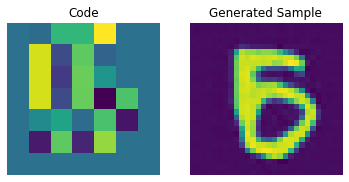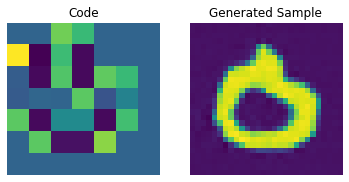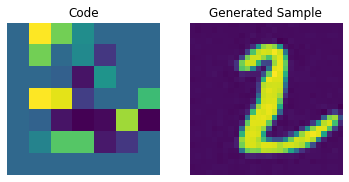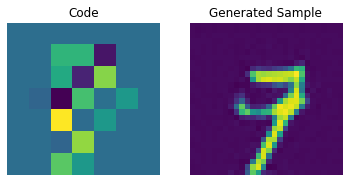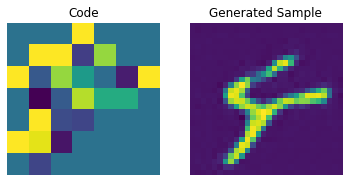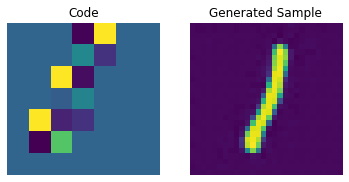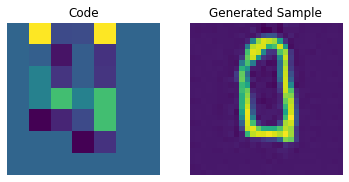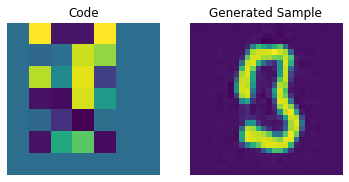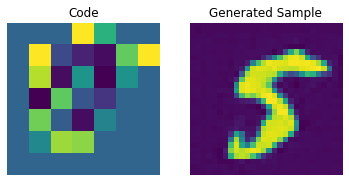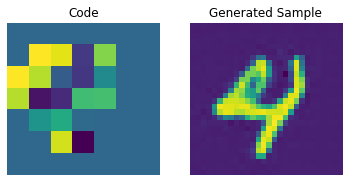We can enhance the quality of these generated samples by tweaking the PixelCNN.